# Distributions

Probability Density Function For a continuous function, the probability density function (pdf) is the probability that the variate has the value x. Since for continuous distributions the probability at a single point is zero, this is often expressed in terms of an integral between two points.

∫baf(x)dx=Pr[a≤X≤b]

For a discrete distribution, the pdf is the probability that the variate takes the value x.

f(x)=Pr[X=x]

The following is the plot of the normal probability density function.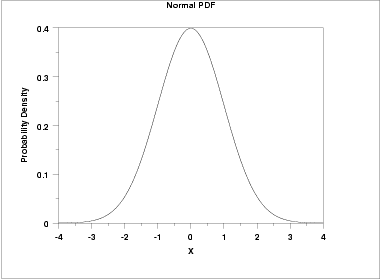Cumulative Distribution Function The cumulative distribution function (cdf) is the probability that the variable takes a value less than or equal to x. That is

F(x)=Pr[X≤x]=α

For a continuous distribution, this can be expressed mathematically as

F(x)=∫x−∞f(μ)dμ

For a discrete distribution, the cdf can be expressed as

F(x)=∑xi=0f(i)

The following is the plot of the normal cumulative distribution function.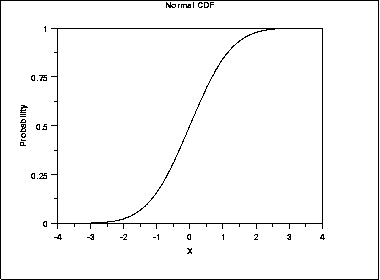The horizontal axis is the allowable domain for the given probability function. Since the vertical axis is a probability, it must fall between zero and one. It increases from zero to one as we go from left to right on the horizontal axis.
Percent Point Function The percent point function (ppf) is the inverse of the cumulative distribution function. For this reason, the percent point function is also commonly referred to as the inverse distribution function. That is, for a distribution function we calculate the probability that the variable is less than or equal to x for a given x. For the percent point function, we start with the probability and compute the corresponding x for the cumulative distribution. Mathematically, this can be expressed as

Pr[X≤G(α)]=α

or alternatively

x=G(α)=G(F(x))

The following is the plot of the normal percent point function.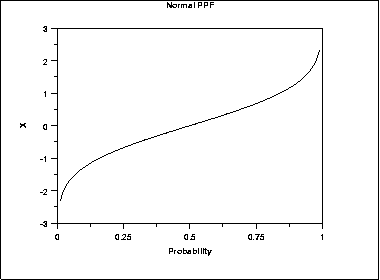Since the horizontal axis is a probability, it goes from zero to one. The vertical axis goes from the smallest to the largest value of the cumulative distribution function.
Hazard Function The hazard function is the ratio of the probability density function to the survival function, S(x).

h(x)=f(x)S(x)=f(x)1−F(x)

The following is the plot of the normal distribution hazard function.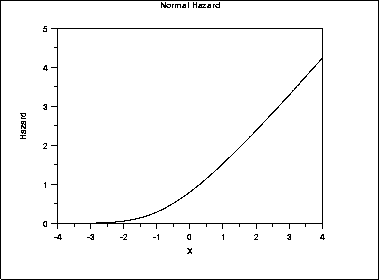Hazard plots are most commonly used in reliability applications. Note that Johnson, Kotz, and Balakrishnan refer to this as the conditional failure density function rather than the hazard function.
Cumulative Hazard Function The cumulative hazard function is the integral of the hazard function.

H(x)=∫x−∞h(μ)dμ

This can alternatively be expressed as

H(x)=−ln(1−F(x))

The following is the plot of the normal cumulative hazard function.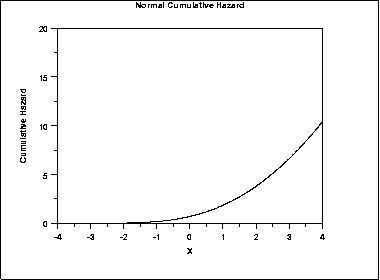Cumulative hazard plots are most commonly used in reliability applications. Note that Johnson, Kotz, and Balakrishnan refer to this as the hazard function rather than the cumulative hazard function.
Survival Function Survival functions are most often used in reliability and related fields. The survival function is the probability that the variate takes a value greater than x.

S(x)=Pr[X>x]=1−F(x)

The following is the plot of the normal distribution survival function.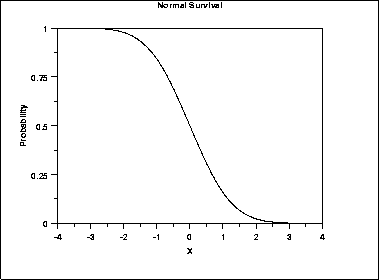For a survival function, the y value on the graph starts at 1 and monotonically decreases to zero. The survival function should be compared to the cumulative distribution function.
Inverse Survival Function Just as the percent point function is the inverse of the cumulative distribution function, the survival function also has an inverse function. The inverse survival function can be defined in terms of the percent point function.

Z(α)=G(1−α)

The following is the plot of the normal distribution inverse survival function.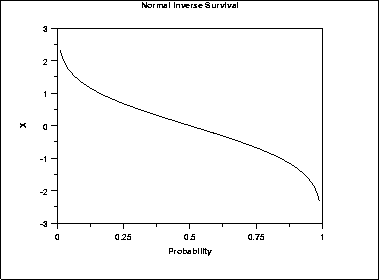As with the percent point function, the horizontal axis is a probability. Therefore the horizontal axis goes from 0 to 1 regardless of the particular distribution. The appearance is similar to the percent point function. However, instead of going from the smallest to the largest value on the vertical axis, it goes from the largest to the smallest value.